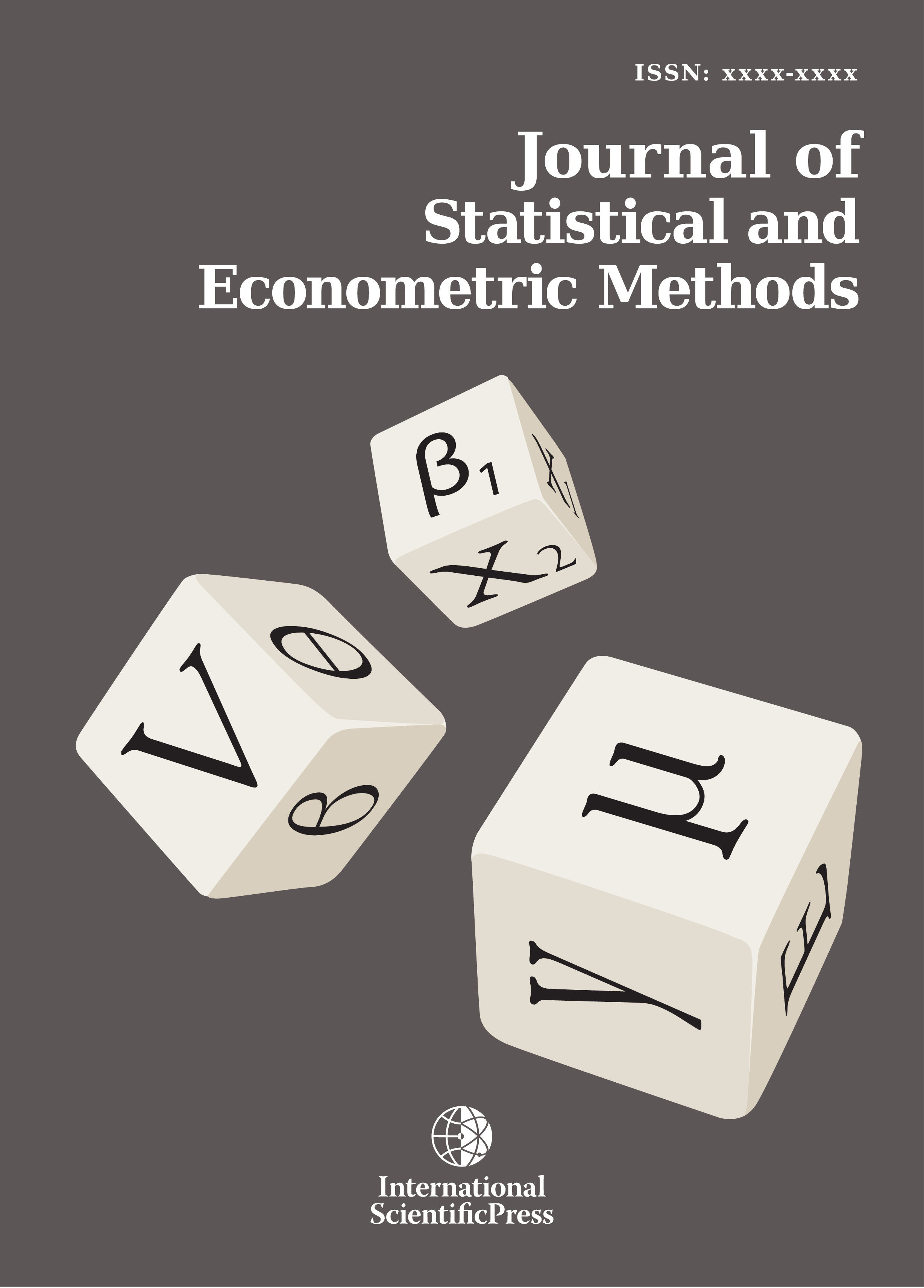# Journal of Statistical and Econometric Methods

#### Omitted Variables Bias in Regime-Switching Models with Slope-Constrained Estimators: Evidence from Monte Carlo Simulations

•[ Download ]
• Times downloaded: 1314
• Abstract

In a recent study, Beccarini  showed that one can eliminate or reduce the bias in OLS regression estimators caused by an omitted polychotomous variable by estimating a regime-switching model. If the missing polychotomous variable assumes K values, then elimination or reduction of the bias requires the estimation of a K-component mixture model. In his Monte Carlo simulations, however, the slope of the parameter of interest is estimated once for each of the K components. After discussing problems associated with multiple estimates of the parameter of interest, this paper extends Beccarini’s Monte Carlo analysis to include the slope-constrained estimator obtained by using the EM algorithm of Bartolucci and Scaccia . We find a small gain in efficiency with the slope-constrained estimator and that the weighted-average estimator in Beccarini  produces a large number of rejections of the true null hypothesis of a single slope when the components are not widely separated.ISSN: 2241-0376 (Online)
2241-0384 (Print)